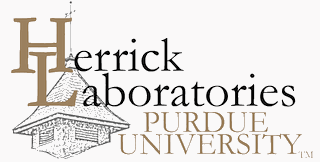## International Refrigeration and Air Conditioning Conference

2190

2012

#### Keywords

modeling, refrigeration, air conditioning, equation-based, block-based

#### Abstract

Transient modeling methods can also be classified into two categories based on equation specifications. The first category of methods are non-equation based methods, in which one has to describe the underlying physics with fundamental equations, formulate residual equations and provide an algorithm to solve the underlying nonlinear equations. The second category of methods are equation based methods, wherein the engineer only has to specify the underlying physics, that holds for each time step, in the form of equations and use an established modeling platform or a solver which will handle the formulation and solution of underlying nonlinear differential algebraic equations. There are two modeling platforms that are widely emerging as an industry standard for transient modeling and control algorithm development. First set of platforms are based on the Modelica® language and the second set are based on MATLAB®. Three commercially available modeling environments based on these platforms are chosen for comparison in this study. In this paper, a transient system model for a simple vapor compression cycle is developed in the three environments (Modelica®, Simulink® and SimScapeTM). The heat exchanger models are based on the finite volume method. The compressor model is an efficiency-based model. The expansion device is modeled using an empirical correlation. Three tools demonstrate quite different modeling concepts. Comparison of simulation results obtained by three tools shows good agreement, but the computation time varies significantly due to underlying model reduction and solution scheme used.

COinS